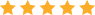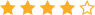## Fractional differential equations & symbolic derivatives and integrals: Power series solutions of fractional differential equations & the nth derivatives and integrals

### Mhenni Benghorbal

0 avg rating
( 0 ratings by Goodreads )View all copies of this ISBN edition:

Fractional differential equations (FDEs) are differential equations having fractional derivatives instead of integer derivatives. For completeness, Chapter 2 is an introduction to fractional derivatives and their definitions including a new idea for the fractional calculus which is the idea of finding the fractional derivative of a function f(x) through its power series. Chapter 3 gives some definitions, classifications, and preliminary theories on the subject of FDEs. Chapter 4 covers the subject of some special functions that have been used in this book. Chapter 5 is devoted to solving some FDEs by constructing compatible bases for their corresponding Fractional differential operators, and then using these bases to approximate the solution using the power series method. The other main part in this work is the subject of finding the nth derivatives and integrals. The problem can be stated as follows: Given a function f(x), can one find a function f(n; x) that gives the nth derivative of f(x) or the nth integral at any point x? This is the subject of Chapter 6 and 7 which has led to finding unified formulas for the nth derivatives and integrals.

"synopsis" may belong to another edition of this title.

The author graduated from Garyounis University (Benghazi, Libya) with a Bs.c in Mathematics in 1989. He got his Ms.c in mathematics from Concordia University (Montreal, Canada) in 1999. He earned his Ph.D in applied mathematics from University of Western Ontario (London, Ontario, Canada) in 2004. Since then he has been holding research positions.

"About this title" may belong to another edition of this title.

Buy New View Book
List Price: US\$ 72.00
US\$ 49.90

Shipping: US\$ 6.52
From United Kingdom to U.S.A.

Destination, rates & speeds

## 1.Fractional Differential Equations &amp; Symbolic Derivatives and Integrals

ISBN 10: 3639715306 ISBN 13: 9783639715309
New paperback Quantity Available: > 20
Seller:
Blackwell's
(Oxford, OX, United Kingdom)
Rating[?]

Book Description Scholars' Press 2014-04-22, 2014. paperback. Condition: New. Seller Inventory # 9783639715309

US\$ 49.90
Convert currency
Shipping: US\$ 6.52
From United Kingdom to U.S.A.
Destination, rates & speeds

## 2.Fractional differential equations & symbolic derivatives and integrals

ISBN 10: 3639715306 ISBN 13: 9783639715309
New Quantity Available: 1
Seller:
Rating[?]

Book Description Condition: New. Publisher/Verlag: Scholar&apos;s Press | Power series solutions of fractional differential equations & the nth derivatives and integrals | Format: Paperback | Language/Sprache: english | 229 gr | 220x150x8 mm | 160 pp. Seller Inventory # K9783639715309

US\$ 55.73
Convert currency
Shipping: US\$ 4.29
From Germany to U.S.A.
Destination, rates & speeds

## 3.Fractional Differential Equations and Symbolic Derivatives and Integrals

ISBN 10: 3639715306 ISBN 13: 9783639715309
New Quantity Available: > 20
Print on Demand
Seller:
Books2Anywhere
(Fairford, GLOS, United Kingdom)
Rating[?]

Book Description Scholars' Press, 2014. PAP. Condition: New. New Book. Delivered from our UK warehouse in 4 to 14 business days. THIS BOOK IS PRINTED ON DEMAND. Established seller since 2000. Seller Inventory # LQ-9783639715309

US\$ 56.35
Convert currency
Shipping: US\$ 11.97
From United Kingdom to U.S.A.
Destination, rates & speeds

## 4.Fractional Differential Equations and Symbolic Derivatives and Integrals

ISBN 10: 3639715306 ISBN 13: 9783639715309
New Quantity Available: > 20
Print on Demand
Seller:
Paperbackshop-US
(Wood Dale, IL, U.S.A.)
Rating[?]

Book Description Scholars' Press, 2014. PAP. Condition: New. New Book. Shipped from US within 10 to 14 business days. THIS BOOK IS PRINTED ON DEMAND. Established seller since 2000. Seller Inventory # IQ-9783639715309

US\$ 68.23
Convert currency
Shipping: US\$ 3.99
Within U.S.A.
Destination, rates & speeds

## 5.Fractional differential equations & symbolic derivatives and integrals

ISBN 10: 3639715306 ISBN 13: 9783639715309
New Taschenbuch Quantity Available: 1
Seller:
BuchWeltWeit Inh. Ludwig Meier e.K.
Rating[?]

Book Description SPS Apr 2014, 2014. Taschenbuch. Condition: Neu. Neuware - Fractional differential equations (FDEs) are differential equations having fractional derivatives instead of integer derivatives. For completeness, Chapter 2 is an introduction to fractional derivatives and their definitions including a new idea for the fractional calculus which is the idea of finding the fractional derivative of a function f(x) through its power series. Chapter 3 gives some definitions, classifications, and preliminary theories on the subject of FDEs. Chapter 4 covers the subject of some special functions that have been used in this book. Chapter 5 is devoted to solving some FDEs by constructing compatible bases for their corresponding Fractional differential operators, and then using these bases to approximate the solution using the power series method. The other main part in this work is the subject of finding the nth derivatives and integrals. The problem can be stated as follows: Given a function f(x), can one find a function f(n; x) that gives the nth derivative of f(x) or the nth integral at any point x This is the subject of Chapter 6 and 7 which has led to finding unified formulas for the nth derivatives and integrals. 160 pp. Englisch. Seller Inventory # 9783639715309

US\$ 60.51
Convert currency
Shipping: US\$ 19.39
From Germany to U.S.A.
Destination, rates & speeds

## 6.Fractional differential equations & symbolic derivatives and integrals

ISBN 10: 3639715306 ISBN 13: 9783639715309
New Taschenbuch Quantity Available: 1
Seller:
Rheinberg-Buch
Rating[?]

Book Description SPS Apr 2014, 2014. Taschenbuch. Condition: Neu. Neuware - Fractional differential equations (FDEs) are differential equations having fractional derivatives instead of integer derivatives. For completeness, Chapter 2 is an introduction to fractional derivatives and their definitions including a new idea for the fractional calculus which is the idea of finding the fractional derivative of a function f(x) through its power series. Chapter 3 gives some definitions, classifications, and preliminary theories on the subject of FDEs. Chapter 4 covers the subject of some special functions that have been used in this book. Chapter 5 is devoted to solving some FDEs by constructing compatible bases for their corresponding Fractional differential operators, and then using these bases to approximate the solution using the power series method. The other main part in this work is the subject of finding the nth derivatives and integrals. The problem can be stated as follows: Given a function f(x), can one find a function f(n; x) that gives the nth derivative of f(x) or the nth integral at any point x This is the subject of Chapter 6 and 7 which has led to finding unified formulas for the nth derivatives and integrals. 160 pp. Englisch. Seller Inventory # 9783639715309

US\$ 60.51
Convert currency
Shipping: US\$ 19.39
From Germany to U.S.A.
Destination, rates & speeds

## 7.Fractional Differential Equations Symbolic Derivatives and Integrals (Paperback)

Published by Scholars Press, United States (2014)
ISBN 10: 3639715306 ISBN 13: 9783639715309
New Paperback Quantity Available: 1
Seller:
The Book Depository EURO
(London, United Kingdom)
Rating[?]

Book Description Scholars Press, United States, 2014. Paperback. Condition: New. Language: English . Brand New Book. Fractional differential equations (FDEs) are differential equations having fractional derivatives instead of integer derivatives. For completeness, Chapter 2 is an introduction to fractional derivatives and their definitions including a new idea for the fractional calculus which is the idea of finding the fractional derivative of a function f(x) through its power series. Chapter 3 gives some definitions, classifications, and preliminary theories on the subject of FDEs. Chapter 4 covers the subject of some special functions that have been used in this book. Chapter 5 is devoted to solving some FDEs by constructing compatible bases for their corresponding Fractional differential operators, and then using these bases to approximate the solution using the power series method. The other main part in this work is the subject of finding the nth derivatives and integrals. The problem can be stated as follows: Given a function f(x), can one find a function f(n; x) that gives the nth derivative of f(x) or the nth integral at any point x? This is the subject of Chapter 6 and 7 which has led to finding unified formulas for the nth derivatives and integrals. Seller Inventory # KNV9783639715309

US\$ 90.76
Convert currency
Shipping: US\$ 3.98
From United Kingdom to U.S.A.
Destination, rates & speeds

## 8.Fractional differential equations & symbolic derivatives and integrals : Power series solutions of fractional differential equations & the nth derivatives and integrals

ISBN 10: 3639715306 ISBN 13: 9783639715309
New Taschenbuch Quantity Available: 1
Print on Demand
Seller:
AHA-BUCH GmbH
(Einbeck, Germany)
Rating[?]

Book Description SPS Apr 2014, 2014. Taschenbuch. Condition: Neu. This item is printed on demand - Print on Demand Neuware - Fractional differential equations (FDEs) are differential equations having fractional derivatives instead of integer derivatives. For completeness, Chapter 2 is an introduction to fractional derivatives and their definitions including a new idea for the fractional calculus which is the idea of finding the fractional derivative of a function f(x) through its power series. Chapter 3 gives some definitions, classifications, and preliminary theories on the subject of FDEs. Chapter 4 covers the subject of some special functions that have been used in this book. Chapter 5 is devoted to solving some FDEs by constructing compatible bases for their corresponding Fractional differential operators, and then using these bases to approximate the solution using the power series method. The other main part in this work is the subject of finding the nth derivatives and integrals. The problem can be stated as follows: Given a function f(x), can one find a function f(n; x) that gives the nth derivative of f(x) or the nth integral at any point x This is the subject of Chapter 6 and 7 which has led to finding unified formulas for the nth derivatives and integrals. 160 pp. Englisch. Seller Inventory # 9783639715309